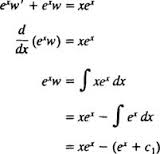Mathematic

# Discuss on Reduction of OrderGeneral purpose of this article is to Discuss on Reduction of Order. Here explain Reduction of Order in Differential Equation perspective in mathematical point of view. Some second‐order equations may be reduced to first‐order equations, rendering them susceptible to the simple methods of solving equations from the first order. The following are three varieties of such second-order equations: Form 1: Second‐order equations with all the dependent variable missing. Form 2: Second‐order nonlinear equations with all the independent variable missing. Form 3: Second‐order homogeneous linear equations where one solution is known. Here explain three particular types with mathematical equation.